# Zero Crossing Detection Circuits Examples

Zero crossing detection circuit comprises two main electronics components. One is an operational amplifier and the second one is passive electronic components such as resistors, capacitors. It detects a zero voltage reference point of the AC signal or a sine wave that is a zero crossing point of the AC signal. The operational amplifier (Op-amp) is the pivotal active component of this circuit. We operate op-amp as a comparator to compare zero voltage reference signals with the AC signal.

## Op-amp as a comparator for zero crossing circuits

Now we will begin with the introduction of an op-amp. How do we use it as a comparator? Because it is the main component of a zero crossing detector circuit. We cannot understand its working without having full command over op-amp.

### Op-amp Introduction

Operational amplifier or in short op-amp is a workhorse of all analog electronics circuits. It is a type of amplifier. Because any amplifier is an electronic circuit that amplifies the input signal to higher or lower values. Similarly, op-amp can also perform amplification. But we can also use it for other purposes i.e. as a comparator, voltage summation, differentiator, integrator, filters, and instrumentation.

### How did it work?

It has two input pins i.e. inverting input and a non-inverting input and one output pin. Power supply pins define the operating voltage range such as Vss+ and Vss-. We can attain high gain through feedback resistive network circuit. But in this ZCD tutorial, we will examine its working as a comparator.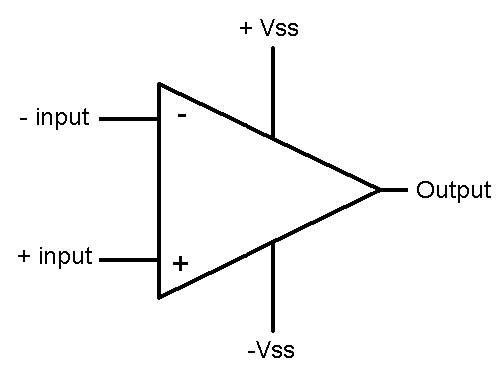### Op-amp as a comparator

The comparator circuit is similar to a traditional amplifier. But it compares inverting and noninverting input signals and produces a digital output. When we use an op-amp as a comparator, it provides output in digital form. Output will be either digital high or digital low. Output signal depends on which input signal is high.

Now let’s look at an example. As this diagram depicts,  if the voltage at the non-inverting input pin is greater than the inverting input, the output of the comparator will be digital high.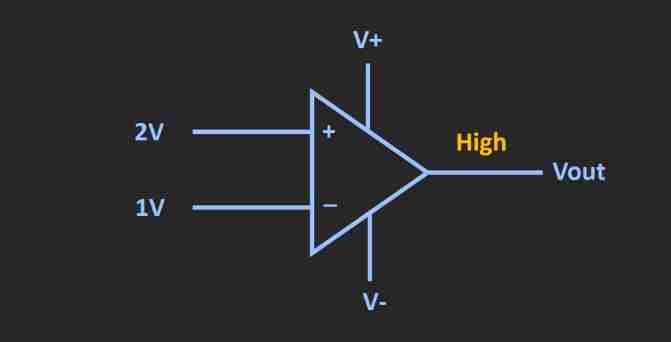Similarly, if the magnitude of the voltage at a non-inverting input pin is less than the inverting pin, the output will be digital low as this circuit diagram depicts.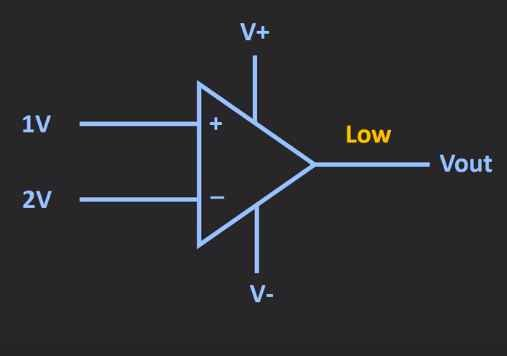## Zero crossing detector examples using op-amp

In the previous section, we understand the working of op-amp as a comparator. We will apply those concepts to design zero crossing detector circuits using operational amplifiers. We also refer them as a sine wave to sqaure wave converter circuits.

Zero crossing detector circuit is similar to a comparator but one of the input pins connects with a ground terminal. We provide zero voltage reference to one of the inputs pins. Because we want to find zero crossings of a sine wave. Whenever a sine wave makes the transition from zero reference voltage, we will get a digital high output. Hence, ZCD detects the zero voltage point of an AC signal. Based on which input pin we connect with a zero voltage reference, we classify it into two types.

### Inverting ZCD Example

In inverting type ZCD, zero crossing detection circuit, we connect zero voltage reference with a noninverting input pin as shown in this diagram. This circuit represents a zero crossing detector circuit using LM741 op-amp. In this configuration, we apply signal voltage to the inverting terminal and zero voltage reference signal to the noninverting input. 2N2222 NPN transistor shifts the voltage level to a digital signal.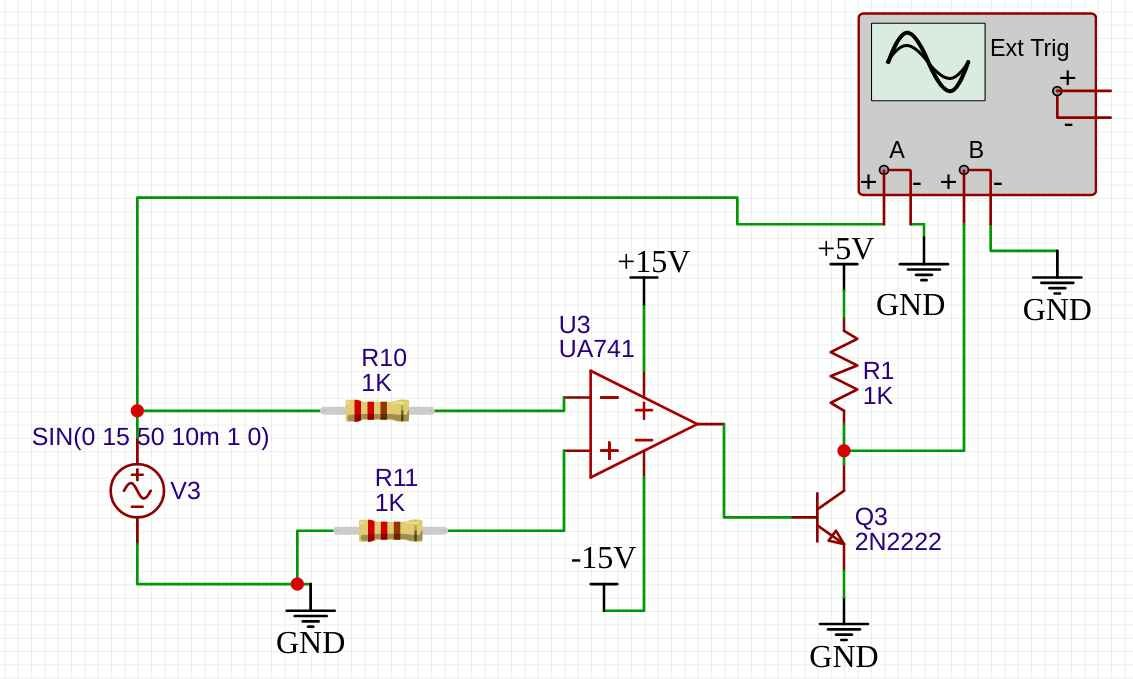The simulation result diagram represents an output signal from a transistor pin.

• During a positive half cycle of the sine wave,  Vin – > Vin + ( that is ground) so the output will be equal to -15 volts, but transistor will remain off and we will get a digital high signal at the transistor collector terminal.
• Similarly, after the transition from positive to the negative cycle, zero reference voltage Vin+ will become greater than the negative voltage signal, the comparator output will become +15 volts. But it will turn on the transistor. We will get digital low signal at the output.

### Noninverting type ZCD Example

The working of this type is exactly same except input pin connections with Vin+ and Vin- input signals. In a non-inverting zero-crossing detector, the input voltage is applied to the non-inverting terminal and the inverting terminal is grounded to make the reference voltage zero. This is a circuit diagram of noninverting ZCD: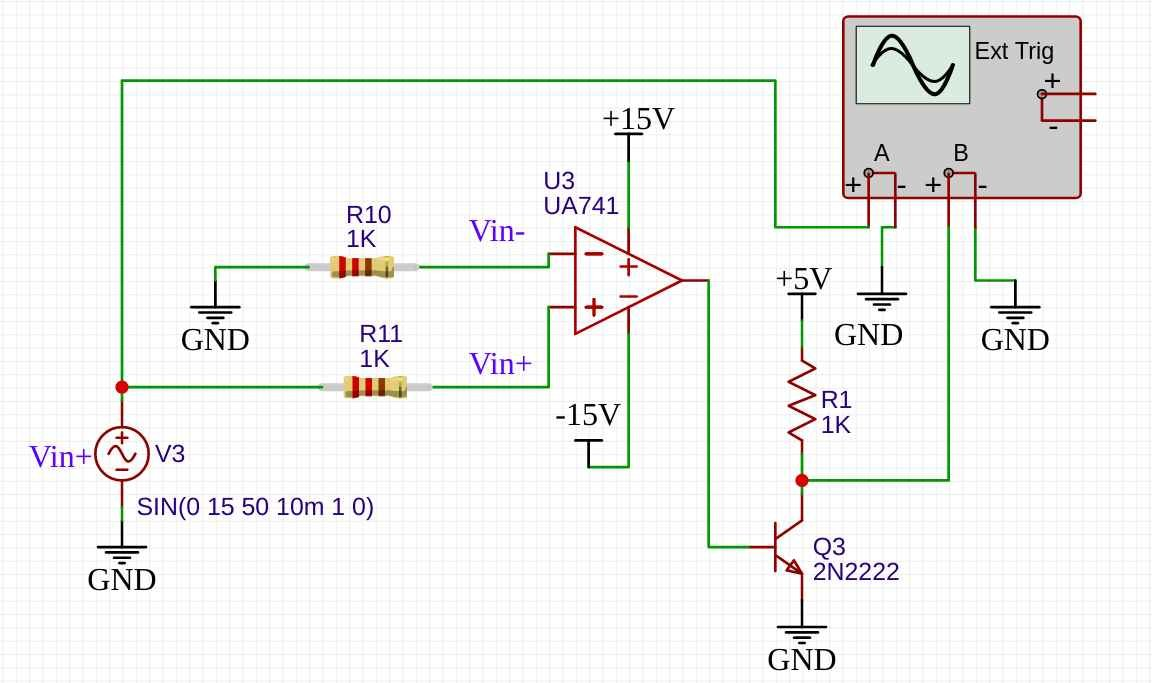• Again we find out the conditions for Vin- reference is equal to zero volts
• when Vin+  is smaller than zero reference voltage Vin- we have output voltage equal to +15 volts. Due to transistor, we get a digital low or zero voltage signal on the collector output terminal. The transistor turns on and makes the collector terminal shorted with the ground.
•  During the positive half cycle of Vin+, the output will be digital high. Because of Vin+ which becomes greater than zero reference voltage Vin-. We get -15 volts at the output pin and transistor remains off.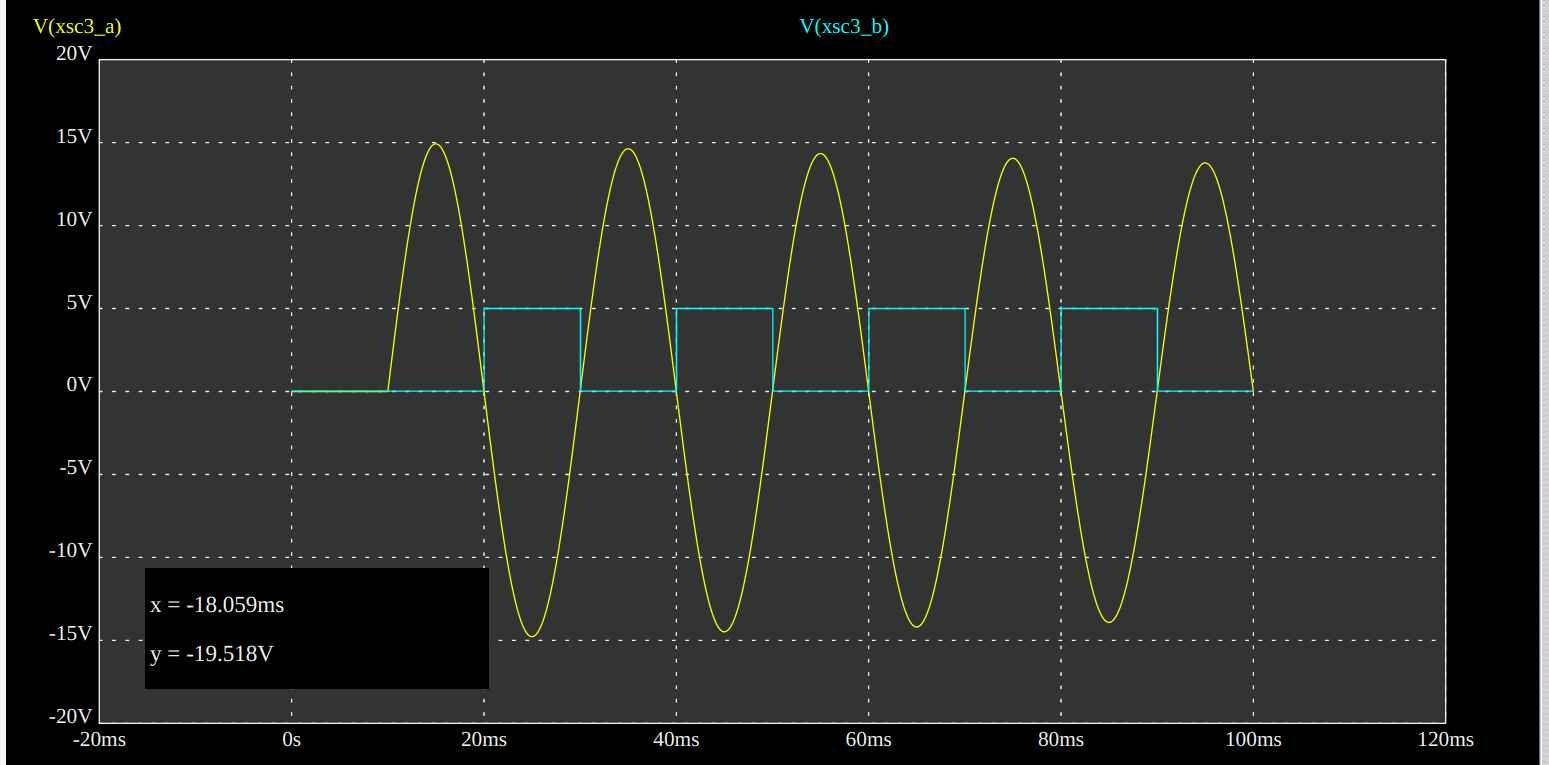## Zero crossing detector with a pulse output

As you can clearly depict from the above simulation results that both outputs signals make the transition from either digital high to low or low to high whenever a sine wave passes zero reference voltage. But after that digital either remain digital high or digital low. Now, what if you want to get a pulse only for a short duration when a sine wave goes from a positive cycle to a negative or negative cycle to a positive cycle?  This circuit provides an output pulse only on cycle transition.

• A full-bridge circuit converts a negative half cycle into a positive half cycle.
• A transistor and resistive network are used as a positive edge detector. It changes the output of operational amplifier into a pulsating output.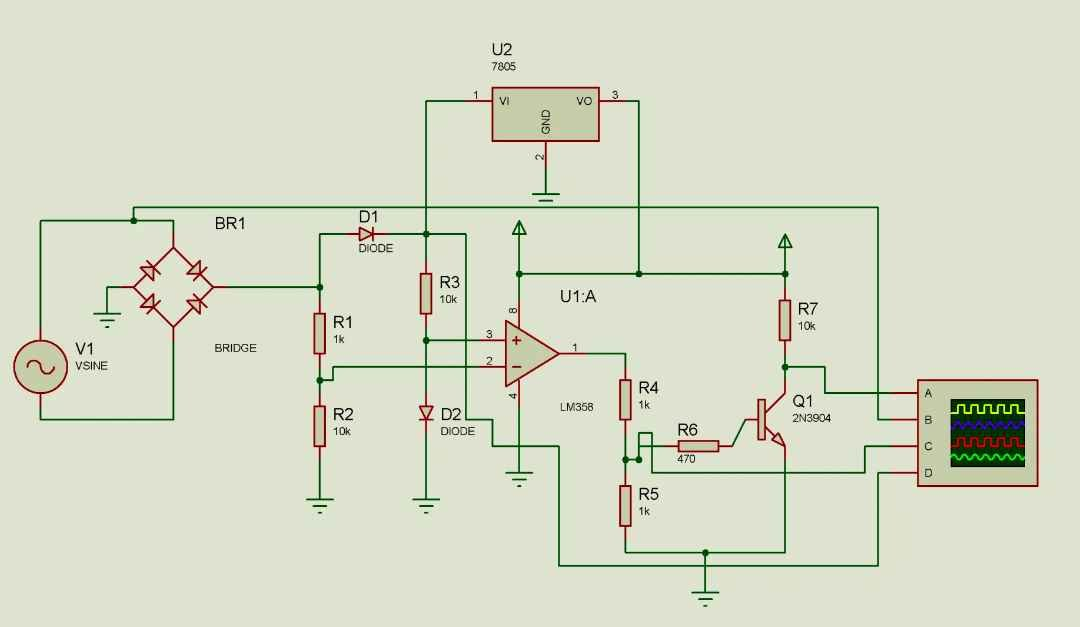### Output diagram

The only difference between previous examples and this example is an edge detection circuit.  We use an LM358 operational amplifier as a comparator. When we want to use a Zero crossing detector with any microcontroller, the square like output is not beneficial for us. This pulse output shows sine wave change from positive to negative cycle and vice versa.

## Zero crossing detection with optocoupler

This circuit provides a pulse output on every zero voltage reference of a sine wave.  4N25 optocoupler remains on during both positive cycles. Because a full-bridge circuit converts a negative cycle into a positive cycle.

• When optocoupler is on, the output will be zero because output will be a ground reference.
• At zero crossing of a sine wave, optocoupler turns off and a pulse of Vcc magnitude appears at the output.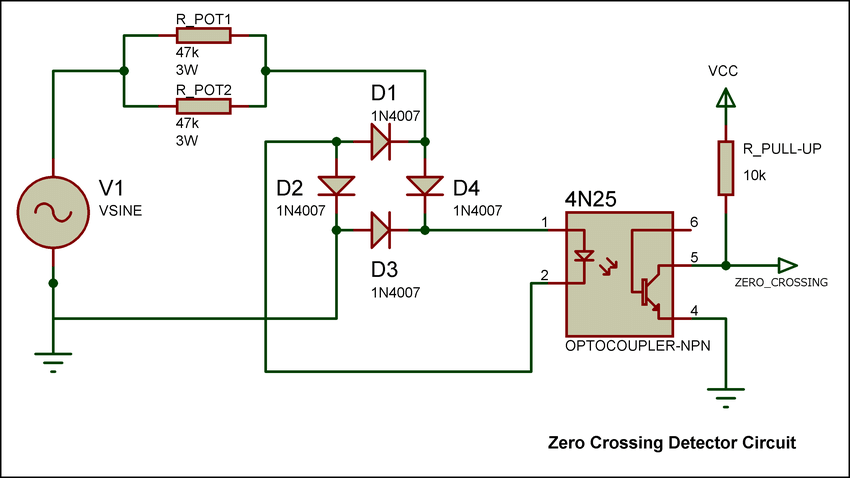## Where to use zero crossing detection?

We usually deploy these circuits with a microcontroller in power electronics projects.  This list provides some practica project examples.

Integrated circuits or IC are also available in the market with built-in zero-crossing features.

## Applications

Zero crossing detection is used in many applications:
• Controlled voltage rectifiers
• Resonant power supplies
• Induction motor speed control and soft starters
• AC power controllers

You can use a simple op-amp for zero-cross detection if you have a little bit of knowledge of operational amplifiers.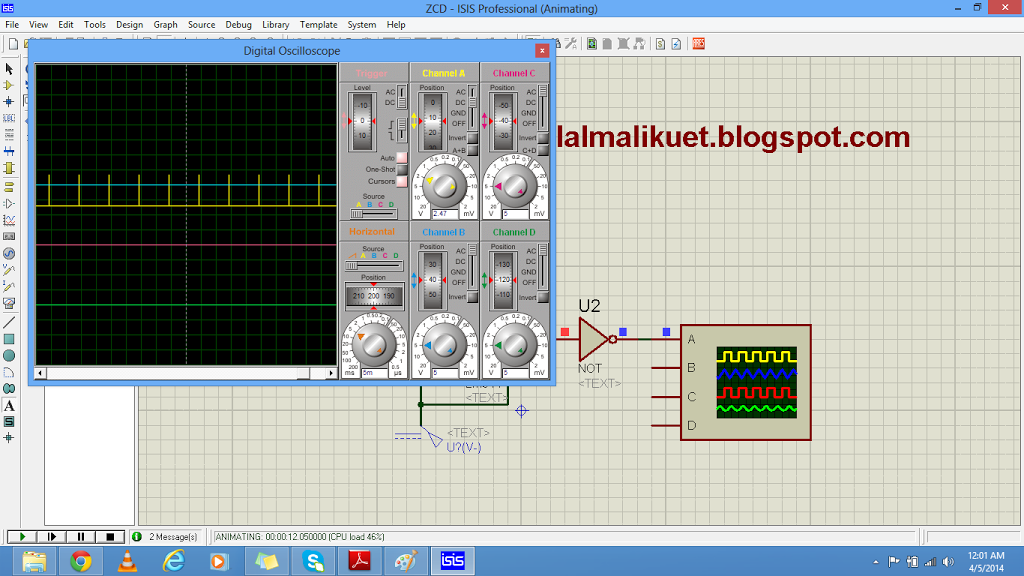### 6 thoughts on “Zero Crossing Detection Circuits Examples”

1. Hi BILAL MALIK it just from Cameroon, i was doing some reached and I came across some of your mini projects that you have design. I’m a final year student in the university of Bamenda in Cameroon and was wandering if we can work together regathering my end of year project.